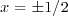D.1 REVIEW OF RIEMANN INTEGRAL

The Riemann integral is covered in introductory calculus courses, and is the integral used in Chapters 3 and 4 on random variables. Consider the function y = g(x) depicted in Figure D.1 which is continuous and bounded on [a, b]. Note that in general an integrable function need not be continuous. Differentiable functions form a subset of continuous functions, and continuous functions form a subset of integrable functions. Later we consider integrals of functions that are not continuous.

FIGURE D.1 Function y = g(x) partitioned on the x-axis into subintervals on [a, b]. Light-shaded rectangles comprise the lower Riemann sum. Light-plus-dark-shaded rectangles comprise the upper Riemann sum.Example D.1. The rectangle function can be written as rect(x) = u(x + 1/2)−u(x−1/2) where u(x) is the unit-step function. It is clear that rect(x) is not differentiable or continuous at, but is integrable on [−1/2, 1/2]: it has unit area. The triangle function tri(x) = (1−|x|)rect(x/2) is continuous and integrable on [−1, 1], but is not differentiable at x = 0.

Observe in Figure D.1 that [a, b] on the x-axis has been partitioned into a collection of subintervals {[xn, xn + 1]} for . Let be a point in the nth subinterval [xn, xn + 1]. The mesh of the partition is ...

Get Probability, Random Variables, and Random Processes: Theory and Signal Processing Applications now with the O’Reilly learning platform.

O’Reilly members experience books, live events, courses curated by job role, and more from O’Reilly and nearly 200 top publishers.